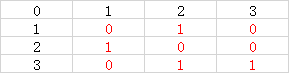# 题目描述：

Alice和Bob在玩一个游戏，游戏是在一个N*N的矩阵上进行的，每个格子上都有

2
2
2 4
6 8
3
5 4 2
1 5 9
7 3 8

L
W

100%数据满足

1<=N<=1000

1<=T<=5

30%数据满足

1<=N<=5

50%数据满足

1<=N<=100

70%数据满足

1<=N<=500

# 思路：

1、用a数组存原矩阵，用b数组存原矩阵“列“”的前缀和，用c数组存原矩阵“行”的前缀和，方便快速判断某一列或某一行可不可以删除
2、从右下角开始模拟删除，如果当前行或当前列可以删除，累加删除次数，否则return
3、如果删除次数是奇数，则Alice必输，如果是偶数则必赢

#### 50分代码：

``````#include<bits/stdc++.h>
using namespace std;
long long t,n,a,b,c,pd=1;
void search(int i,int j)
{

if (b[i][j]%2!=0&&c[i][j]%2!=0||i==0||j==0) return;
if (b[i][j]%2==0) pd++,search(i-1,j);
if (c[i][j]%2==0) pd++,search(i,j-1);
}
int main()
{

pd=1;
cin>>t;
for (int k=1;k<=t;k++)
{

cin>>n;
for (int i=0;i<=n;i++)
for (int j=0;j<=n;j++) b[i][j]=0,c[i][j]=0;
for (int i=1;i<=n;i++)
for (int j=1;j<=n;j++)
{

cin>>a[i][j];
b[i][j]=b[i][j-1]+a[i][j];
c[i][j]=c[i-1][j]+a[i][j];
}
search(n,n);
if (pd%2!=0) cout<<"L"<<endl;
else cout<<"W"<<endl;
}
return 0;
}
``````

f[i][j]表示从右下角为i,j坐标开始删除Alice先手的输赢状况，1为必赢，0为必输，我们只要把对方置为必输状况就可以赢了，判断当前输赢状况分三种情况
1、当f[i][j]左边和上边都是必赢时，无论当前怎么删，到了对方就是必赢，所以我方必输
2、当只有一个是必赢时，判断可否删除必赢的那一行或列，将对手置于必输状况，如果可以删除，那么当前位置就必赢
3、当两个都必输时，任意删除偶数行或偶数列，都可以将对手置于必输状况，如果都删不了，那么就必输#### AC代码：

``````#include<bits/stdc++.h>
using namespace std;
int t,n,a,b,c,f;
int main()
{

cin>>t;
for (int k=1;k<=t;k++)
{

cin>>n;
for (int i=0;i<=n;i++)
for (int j=0;j<=n;j++) f[i][j]=0,b[i][j]=0,c[i][j]=0;
for (int i=1;i<=n;i++)
for (int j=1;j<=n;j++)
{

cin>>a[i][j];
b[i][j]=b[i][j-1]+a[i][j];
c[i][j]=c[i-1][j]+a[i][j];//前缀和
}
if (a%2==0) f=1; else f=0; //初始化
for (int i=1;i<=n;i++)
for (int j=1;j<=n;j++)
{

if (f[i-1][j]==0&&f[i][j-1]==0)
if (b[i][j]%2==0||c[i][j]%2==0) f[i][j]=1;//只要前两个都是零并且可以删，那么这个位置必赢
if (f[i-1][j]==0&&f[i][j-1]==1)
if (b[i][j]%2==0) f[i][j]=1;
if (f[i-1][j]==1&&f[i][j-1]==0)
if (c[i][j]%2==0) f[i][j]=1;  //前两个一个0一个1，对应的可以删就必赢
}
if (f[n][n]==1) cout<<"W"<<endl; //最后是1，必赢
else cout<<"L"<<endl;
}
return 0;
}
``````

### vue之vuex的五个属性_jh1906338271的博客-程序员秘密

1.官方解释Vuex是一个专为Vue.js应用程序开发的状态管理模式。然后Vuex里面有五个特别重要的属性，分别是state，mutations，actions，getters，modules。2.state放置状态相关的信息，vue是使用单一状态树的，也就是单一数据源，也就是说我们的state只能有一个3.mutationsmutations其实就相当于我们vue里面的methods...

### bzoj千题计划188：bzoj1923: [Sdoi2010]外星千足虫 （高斯—若尔当消元法解异或方程组）..._weixin_30322405的博客-程序员秘密

http://www.lydsy.com/JudgeOnline/problem.php?id=1923#include&lt;cstdio&gt;#include&lt;cstring&gt;#include&lt;iostream&gt;#include&lt;bitset&gt;using namespace std;int n,m;bitset&lt;...

### html之如何让多个并列的div居中显示_多个div并排居中_Quincy379的博客-程序员秘密

<div id="testContainer"> <div><img src="1.png"></div> <div><img src="1.png"></div> <div><img src="1.png"></div> <div><img src="1.png"></div> <div><img src="1.png"></div> <div><i